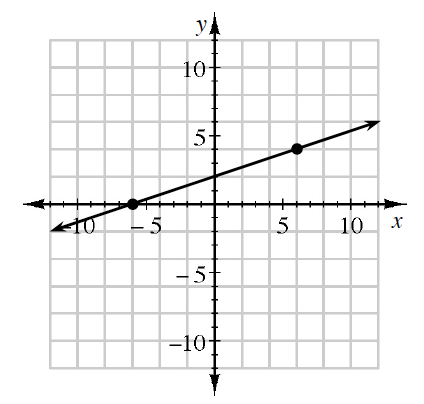### Home > CC4 > Chapter 2 > Lesson 2.1.2 > Problem2-18

2-18.

Calculate the slope of the line shown on the graph at right. Homework Help ✎

$\text{Slope }=\frac{\Delta y}{\Delta x}=\frac{\text{rise}}{\text{run}}$

You can use the two points on the line.
One of them is$(−6, 0)$.